Next: Constraints for the vector-isoscalar Up: Continuity equations in the Previous: Constraints for the scalar-isoscalar

## Constraints for the scalar-isovector channel

Validity of the CE for the scalar-isovector density, Eq. (30) for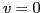and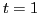, imposes through Eq. (48) certain specific constraints on the coupling constants of the functional. At second order we obtain,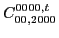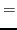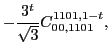(53)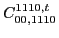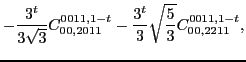(54)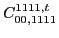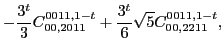(55)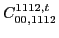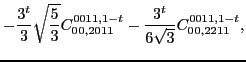(56)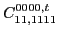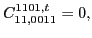(57)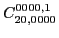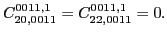(58)

Constraints in Eqs. (53)-(56) connect the isoscalar and isovector coupling constants. The numerical coefficients of the corresponding linear combinations are the same as those for the scalar-isoscalar CE, see Eqs. (C1)-(C4) of Ref. [Carlsson et al.(2008)Carlsson, Dobaczewski, and Kortelainen], apart from factors of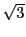explained before Eq. (36). However, conditions for the scalar-isoscalar CE keep the coupling constants in the two isospin channels disconnected. Moreover, in the scalar-isovector channel the spin-orbit coupling constants must vanish, Eq. (57), along with the isovector surface coupling constants, Eq. (58). On the other hand, the corresponding isoscalar surface coupling constants,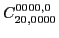,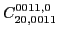, and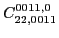, are left unrestricted.

For the fourth and sixth orders, analogous constraints are presented in Appendix A.Next: Constraints for the vector-isoscalar Up: Continuity equations in the Previous: Constraints for the scalar-isoscalar
Jacek Dobaczewski 2011-11-11